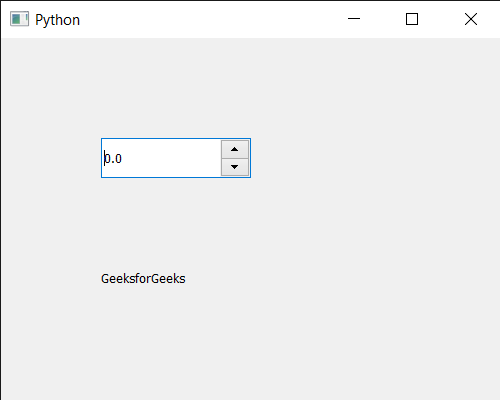Related Articles

# PyQt5 QDoubleSpinBox – Setting Line Edit to it

• Last Updated : 28 Jul, 2020

In this article we will see how we can set the line edit to the QDoubleSpinBox. Line edit is the white space which receives the input and shows the current value of the double spin box, we can change the line edit object any time. Line edit is the main component of the double spin box the default line edit is made such that it receives only float values.

In order to do this we will use `setLineEdit` method with the double spin box object.

Syntax : dd_spin.setLineEdit(lineedit)

Argument : It takes QLineEdit object as argument

Return : It returns None

Below is the implementation

 `# importing libraries``from` `PyQt5.QtWidgets ``import` `*` `from` `PyQt5 ``import` `QtCore, QtGui``from` `PyQt5.QtGui ``import` `*` `from` `PyQt5.QtCore ``import` `*` `import` `sys`` ` ` ` `class` `Window(QMainWindow):`` ` `    ``def` `__init__(``self``):``        ``super``().__init__()`` ` `        ``# setting title``        ``self``.setWindowTitle(``"Python "``)`` ` `        ``# setting geometry``        ``self``.setGeometry(``100``, ``100``, ``500``, ``400``)`` ` `        ``# calling method``        ``self``.UiComponents()`` ` `        ``# showing all the widgets``        ``self``.show()`` ` `    ``# method for components``    ``def` `UiComponents(``self``):`` ` `        ``# creating double spin box``        ``d_spin ``=` `QDoubleSpinBox(``self``)`` ` `        ``# setting geometry to the double spin box``        ``d_spin.setGeometry(``100``, ``100``, ``150``, ``40``)`` ` `        ``# setting decimal precision``        ``d_spin.setDecimals(``1``)`` ` `        ``# step type``        ``step_type ``=` `QAbstractSpinBox.AdaptiveDecimalStepType`` ` `        ``# adaptive step type``        ``d_spin.setStepType(step_type)`` ` `        ``# line edit``        ``lineedit ``=` `QLineEdit(``self``)`` ` `        ``# setting line edit to the double spin box``        ``d_spin.setLineEdit(lineedit)`` ` ` ` `        ``# creating a label``        ``label ``=` `QLabel(``"GeeksforGeeks"``, ``self``)`` ` `        ``# setting geometry to the label``        ``label.setGeometry(``100``, ``200``, ``300``, ``80``)`` ` `        ``# making label multi line``        ``label.setWordWrap(``True``)`` ` ` ` ` ` ` ` ` ` `# create pyqt5 app``App ``=` `QApplication(sys.argv)`` ` `# create the instance of our Window``window ``=` `Window()`` ` `# start the app``sys.exit(App.``exec``())`

Output :Attention geek! Strengthen your foundations with the Python Programming Foundation Course and learn the basics.

To begin with, your interview preparations Enhance your Data Structures concepts with the Python DS Course. And to begin with your Machine Learning Journey, join the Machine Learning – Basic Level Course

My Personal Notes arrow_drop_up